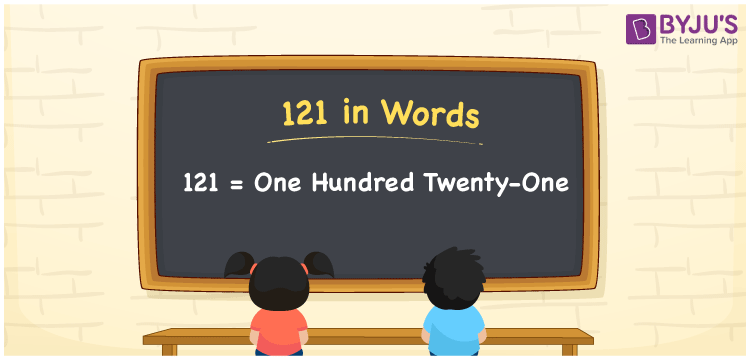# 121 in Words

We can write 121 in words as One hundred twenty-one. If you observed Rs. 121, on a menu card, you may read it as “One hundred twenty-one rupees”. That means to spell the given number or express the numerical quantity, we will use the word form of that particular number. In this article, you will learn how to derive the cardinal number 121 in words using a place value chart.

 121 in words One hundred twenty-one One hundred twenty-one in Numerical Form 121

## 121 in English Words

We generally use the English alphabet to write numbers in words. Thus, we write 121 in English words as “One hundred twenty-one”.## How to Write 121 in Words?

As we can see, the number 121 contains three digits. Thus, we need to create a place value chart with three columns as given below.

 Hundreds Tens Ones 1 2 1

Here, ones = 1, tens = 2, hundreds = 1

The above values can be expanded as:

1 × Hundred + 2 × Ten + 1 × One

= 100 + 20 + 1

= 100 + 21

= One hundred + Twenty-one

= One hundred twenty-one

Therefore, 121 in words = One hundred twenty-one

121 is a natural number that precedes 122 and succeeds 120.

121 in words – One hundred twenty-one

Is 121 an even number? – No

Is 121 an odd number? – Yes

Is 121 a prime number? – No

Is 121 a composite number? – Yes

Is 121 a perfect square number? – Yes

What is the square root of 121? – 11

Is 121 a perfect cube number? – No

## Related Articles

### 121 to 130 in Words

 121 in words = One hundred twenty-one 126 in words = One hundred twenty-six 122 in words = One hundred twenty-two 127 in words = One hundred twenty-seven 123 in words = One hundred twenty-three 128 in words = One hundred twenty-eight 124 in words = One hundred twenty-four 129 in words = One hundred twenty-nine 125 in words = One hundred twenty-five 130 in words = One hundred thirty

## Frequently Asked Questions on 121 in Words

Q1

### How do you write 121 in words?

We can write the number 121 in words as One hundred twenty-one.
Q2

### Express 121.5 in words.

We can express the decimal number 121.5 in words as One hundred twenty-one point five.
Q3

### How to write Rs. 121 in words on a cheque?

On a cheque, we can write the amount Rs. 121 in words as “One hundred twenty-one rupees only”.
Test your Knowledge on 121 in Words